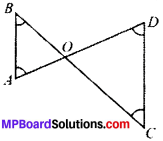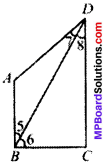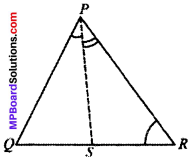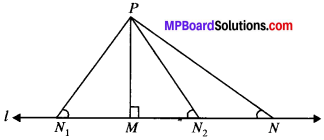# MP Board Class 9th Maths Solutions Chapter 7 Triangles Ex 7.4

## MP Board Class 9th Maths Solutions Chapter 7 Triangles Ex 7.4

Question 1.
Show that in a right angled triangle, the hypotenuse is the longest side.
Solution:
Given
ABC is a right angle A.
To prove:
AC > AB and AC > BCProof:
In ∆ABC
∠B = 90°
∴ ∠A + ∠C = 90° (by ASP)
and so ∠B > ∠A and ∠B > ∠C
AC > BC and AC > AB
(In a A, sides opposite to large angle are always longer).Question 2.
In Fig. given below, sides AB and AC of ∆ABC are extended to points P and Q respectively. Also, ∠PBC < ∠QCB. Show that AC > AB.
Solution:
Given
∠PRC < ∠QCB To prove AC > ABProof:
In ∆ ABC
Exterior angle is equal to sum of two opposite angles
∠PBC = ∠1 + ∠3 and
∠QCB = ∠1 + ∠2
∠QCB > ∠PBC (given)
⇒ ∠1 + ∠2 > ∠1 + ∠3
⇒ ∠2 > ∠3
∴ AC > AB
(∴ In a A, side opposite to larger angle is always longer).

Question 3.
In Fig. below, ∠B < ∠A and ∠C < ∠D. Show that AD < BC.Solution:
Given
∠B < ∠A i.e., ∠A > ∠B
∠C < ∠D i.e., ∠D> ∠C
Proof:
In ∆OCD
∠D > ∠C
OC > OD
(∴ In a ∆, sides opposite to larger angle are always longer) …(1)
In ∆OBA
∠A > AB
OB > OA
(∴ In a A, sides oppositedo larger angle are always longer) …(2)
Adding (1) and (2), we get
OC + OB > OD + OAQuestion 4.
AB and CD are respectively the smallest and longest sides of a quadrilateral ABCD (see Fig. below). Show that ∠A > ∠C and ∠B > ∠D.
Solution:
Given
AB is the smallest siBe and CD is the longest side
To prove:

1. ∠A > ∠C and
2. ∠B > ∠D

Construction:
Join AC1. Proof:
In, ∆ABC
BC > AB (∴ AB is the smallest side) ∠1 > ∠3
(∴ In a ∆ angle opposite to longer side is always larger) …..(1)
In ∆ACD
CD > AD (∴ CD is the largest side) ∠2 > ∠4
(∴ In a ∆ angles opposite to longer side are always larger) …(2)
Adding (1) and (2), we get
∠1 + ∠2 > ∠3 + ∠4
∠A > ∠C

2. To prove: AB > AD
Construction:
Join BD
Proof:
In ∆ABD
AD > AB (AB is the smallest side)
∠5 > ∠7 …(3)
In ∆BCD
CD > BC (CD is the longest side)∠6 > ∠8 …(4)
Adding (3) and (4), we get
∠5 + ∠6 > ∠7 + ∠8
∠B > ∠D

Question 5.
In Fig. below, PR > PQ and PS bisects ∠QPR. Prove that ∠PSR > ∠PSQ.Sol.
Given:
PR > PQ
∠1 = ∠2
To prove:
∠PSR > ∠PSQ
Proof:
In ∆PSQ
∠PSR = ∠1 + ∠Q (EAP)
In ∆PSR
∠PSQ = ∠2 + ∠R (EAP)
= ∠1 + ∠R (∠1 = ∠2)
In ∆PQR
PR > PQ (given)
∠Q > ∠R
(∴ In a ∆, angle opposite to longer side is always larger)
∠Q + ∠l > ∠R + ∠1
∴ ∠PSR > ∠PSQ
(∴ ∠PSR = ∠1 + ∠Q and ∠PSQ = ∠1 + ∠R)Question 6.
Show that of all the line segments drawn from a give point not on it, the perpendicular line segment is the shortest.
Solution:
Given
Let us consider the ∆PMN such that ∠M = 90°
Since, ∠M + ∠N + ∠P = 180° [Sum of angles of a triangle]∠M = 90° [PM ⊥ l]
∠N < ∠M
PM < PN …..(1)
Similarly PM < PN1 …..(2)
PM < PN2 …..(3)
From (1), (2) and (3), we have PM is the smallest line segment drawn from P on the line l.
Thus, the perpendicular segment is the shortest line segment drawn on a line from a point not on it.